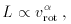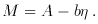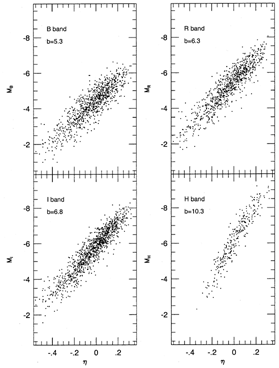### 3. THE TULLY-FISHER RELATION FOR SPIRAL GALAXIES

It has been stated that the Tully-Fisher (TF) relation is the ``workhorse'' of peculiar velocity surveys. One can anticipate a time in the not so distant future when more accurate techniques may supplant it, but for the next few years at least, the TF relation is likely to remain the most widely used distance indicator in cosmic velocity studies. Its role in such studies to date has been, in fact, too large to be reviewed here, and interested readers are referred to Strauss & Willick (1995) Section 7. Several recent developments are discussed in Section 8.

The TF relation is one of the most fundamental properties of spiral galaxies. It is the empirical statement of an approximately power-law relation between luminosity and rotation velocity. Specifically, it is found that(3)

or, using the logarithmic formulation preferred by working astronomers,(4)

In equation (4) M = -2.5 log(L) + const. is the absolute magnitude, and the velocity width parameterlog(2vrot) - 2.5, where vrot is expressed in km s-1, is a useful dimensionless measure of rotation velocity.Figure 2. TF relations in four bandpasses. The absolute magnitudes are given in units such that d = 100.2(m-M) is the galaxy distance in km s-1. Adapted from Strauss & Willick (1995).

An important fact, not always sufficiently appreciated, is that the power law exponentdoes not have a unique value. The details of both the photometric and spectroscopic measurements affect it. A typical result found in contemporary studies is3. The corresponding value of the ``TF slope,'' b, is ~ 7.5. However, slight changes in the details of measurement can result in significant changes in b. This is illustrated in Figure 2, in which TF relations in four bandpasses are plotted. The optical bandpasses (B, R, and I) all represent data from the sample of Mathewson et al. (1992). In each case the slope is < 7. This is because Mathewson et al. defined their velocity widths rather differently than most observers, with the result that small velocity widths are made smaller still relative to other width measurement systems, while large velocity widths are unchanged in comparison with other systems. If one were to transform the Mathewson et al. widths to those of standard systems, one would obtain and I-band TF slope of ~ 7.7, comparable to other I-band samples (Willick et al. 1997). Note, however, that the slope increases steadily from B to I. This reflects a general trend of increasing TF slope toward larger wavelengths, which was noted over a decade ago (Bottinelli et al. 1983). The H-band data come from the compilation of Aaronson et al. (1982), as reanalyzed by Tormen & Burstein (1995) and Willick et al. (1996). The H-band TF slope is considerably greater than its optical counterparts. In part this reflects the trend just noted. However, to a greater extent it is due to the relatively small apertures within which the H-band photometry is done: the TF slope increases as the photometric aperture size is decreased (Willick 1991). Infrared TF samples in which total magnitudes were measured exhibit TF slopes not much in excess of the optical values (Bernstein et al. 1994).

The numerical value of the TF zero point A has no absolute significance in itself, reflecting mainly the photometric system in which the TF measurements are done. Absolute magnitudes measured in different bandpasses can differ numerically by a few magnitudes for a given object. Clearly, this must have no meaning for distance measurements, and it is the zero point that absorbs such differences. For any given measurement system, however, the value of A is highly significant. To see this, consider how the TF relation is used to infer distances and peculiar velocities. Given a measured apparent magnitude m and width parameter, one infers the distance modulus to the galaxy as µ = m - (A - b). The corresponding distance d100.2µ. It follows that an errorA in the TF zero point thus corresponds to a fractional distance error f = 10-0.2A. A Hubble constant inferred from such distances will then be off by a factor f-1. For peculiar velocities, calibration of the TF relation consists in choosing A such that d100.2[m - (A - b)] gives a galaxy's distance in km s-1. There is no requirement that it yield the distance in Mpc. Nonetheless, as mentioned above, zero point calibration errors are still possible. Errors in A produce distances in km s-1 that differ by a fraction f from the true Hubble velocity, with resultant peculiar velocity errorsvp = -fd.

The TF relation has a rich history (cf. Bottinelli et al. 1983) which can not be discussed in any detail here. Its discovery is generally credited to Tully & Fisher (1977), who were the first to suggest a linear correlation between absolute magnitude and log rotation speed. Until the late 1980s, the velocity widths used in TF studies were generally obtained from analysis of the 21 cm line profiles, rather than from direct measurements of rotation curves. Thus, the TF relation was (and to some extent remains) closely associated with 21 cm radio astronomy. There is no inherent connection, however, between the TF relation and the 21 cm line. The TF relation is also closely associated in the minds of many with infrared magnitudes. This is largely due to the pioneering H-band (1.6 µm) TF work of a group headed by the late Marc Aaronson in the late 1970s through the mid-1980s (e.g., Aaronson et al. 1980, 1982, 1986). This group argued that the infrared was better than the optical for TF purposes because infrared magnitudes are less subject to internal and Galactic extinction (Section 3.2), and because they are sensitive mainly to the old stellar population that best traces mass. While these arguments are true at some level, work over the last decade has demonstrated that CCD imaging photometry in red passbands (e.g., R or I) results in TF relations that, empirically, work as well or better than the H-band version (Pierce & Tully 1988, 1992; Willick 1991; Han 1992; Courteau 1992; Bernstein et al. 1994; Willick et al. 1995, 1996).